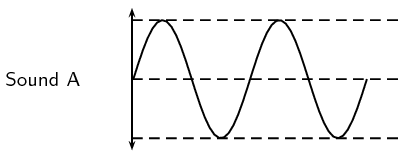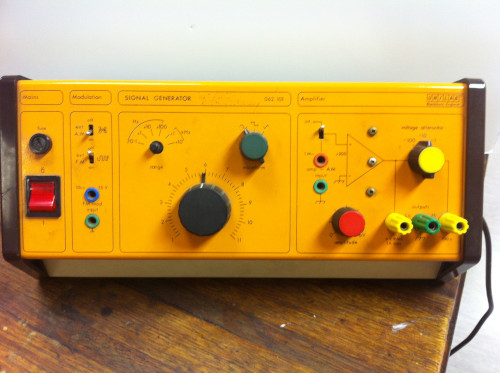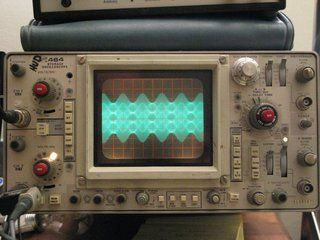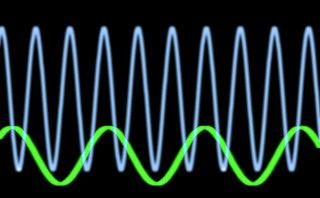Home Practice
For learners and parents For teachers and schools
Textbooks
Full catalogue
Pricing SupportLog in

We think you are located in United States. Is this correct?

# 10.3 Characteristics of a sound wave

## 10.3 Characteristics of a sound wave (ESADD)

Since sound is a wave, we can relate the properties of sound to the properties of a wave. The basic properties of sound are: pitch, loudness and tone.Figure 10.2: Pitch and loudness of sound. Sound B has a lower pitch (lower frequency) than Sound A and is softer (smaller amplitude) than Sound C.

Pitch

The frequency of a sound wave is what your ear understands as pitch. A higher frequency sound has a higher pitch, and a lower frequency sound has a lower pitch. In Figure 10.2 sound A has a higher pitch than sound B. For instance, the chirp of a bird would have a high pitch, but the roar of a lion would have a low pitch.

The human ear can detect a wide range of frequencies. Frequencies from 20 to 20 000 Hz are audible to the human ear. Any sound with a frequency below 20 Hz is known as an infrasound and any sound with a frequency above $$\text{20 000}$$ $$\text{Hz}$$ is known as an ultrasound.

Table 10.2 lists the ranges of some common animals compared to humans.

 lower frequency ($$\text{Hz}$$) upper frequency ($$\text{Hz}$$) Humans $$\text{20}$$ $$\text{20 000}$$ Dogs $$\text{50}$$ $$\text{45 000}$$ Cats $$\text{45}$$ $$\text{85 000}$$ Bats $$\text{20}$$ $$\text{120 000}$$ Dolphins $$\text{0,25}$$ $$\text{200 000}$$ Elephants $$\text{5}$$ $$\text{10 000}$$

Table 10.2: Range of frequencies

temp text

## Range of wavelengths

Using the information given in Table 10.2, calculate the lower and upper wavelengths that each species can hear. Assume the speed of sound in air is $$\text{344}$$ $$\text{m·s^{-1}}$$.

Loudness

The amplitude of a sound wave determines its loudness or volume. A larger amplitude means a louder sound, and a smaller amplitude means a softer sound. In Figure 10.2 sound C is louder than sound B. The vibration of a source sets the amplitude of a wave. It transmits energy into the medium through its vibration. More energetic vibration corresponds to larger amplitude. The molecules move back and forth more vigorously.

The loudness of a sound is also determined by the sensitivity of the ear. The human ear is more sensitive to some frequencies than to others. The volume we receive thus depends on both the amplitude of a sound wave and whether its frequency lies in a region where the ear is more or less sensitive.

## Sound, frequency and amplitude

Textbook Exercise 10.1

Study the following diagram representing a musical note. Redraw the diagram for a note

1. with a higher pitch

2. that is louder

3. that is softer

Solution not yet available

## Comparing sound generating instruments

The size and shape of instruments influences the sounds that they are able to produce. Find some instruments that have different physical characteristics and compare their sounds. You could:

Option 1: Vuvuzelas:

Compare the sounds made by blowing through vuvuzelas of different sizes. You will need to find a few different vuvuzelas. Take turns blowing the different ones, one at a time and record which you think is louder (amplitude), which is of higher pitch (frequency).

Option 2: Tuning forks:

Compare the sounds created by tapping tuning forks of different sizes.

You will need to find a few different tuning forks. Take turns tapping the different ones, one at a time and record which you think is louder (amplitude), which is of higher pitch (frequency).

Option 3: Signal generator and oscilloscope

Use a function generator connected to a speaker to produce sounds of different frequencies and amplitudes and use a microphone connected to an oscilloscope to display the characteristics of the different sounds produced.

Function generator

The function generator allows you to control the loudness and frequency of the sound being produced by the speaker. It will have controls for amplitude and frequency.

A function generatorOscilloscope

The microphone can then pick up the sound and convert it to an electrical signal which can be displayed on the oscilloscope.

The most common oscilloscope controls are for amplitude, frequency, triggering, and channels. Once your teacher has helped you acquire a signal using the correct channel and triggering you will use the amplitude and frequency controls to display the characteristics of the sound being produced.

The amplitude adjustment of an oscilloscope controls how tall a given voltage will appear on the screen. The purpose of this adjustment is that you can see a very large or a very small signal on the same screen.

An oscilloscopeTwo different oscilloscope tracesThe frequency (or time) adjustment of an oscilloscope is how much time will a certain distance across the screen represent. The purpose of this adjustment is to be able to see a very quickly changing or a slowly changing signal on the same screen.

Note:The display of the oscilloscope will show you a transverse wave pattern. This does not mean that sound waves are transverse waves but just shows that the pressure being measured is fluctuating because of a pressure wave.

You will be able to experiment with different amplitudes and frequencies using the function generator and see what impact the changes have on the waveform picked up by the microphone.